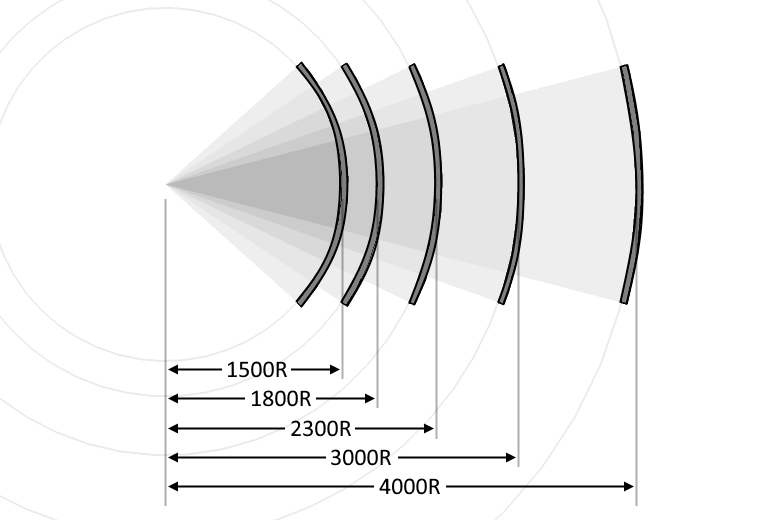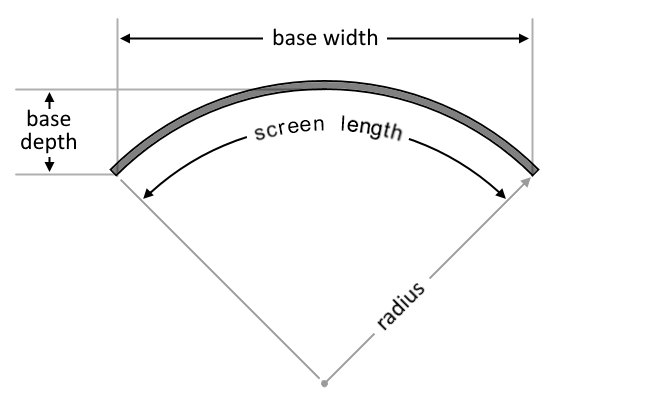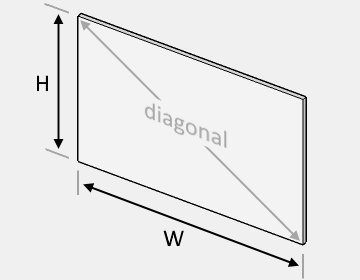# Screen Size Calculator

Created by Hanna Pamuła, PhD candidate and Wojciech Sas, PhD candidate
Reviewed by Bogna Szyk
Last updated: Mar 22, 2022

The screen size calculator can help you in determining the screen dimensions. Have you ever wondered what does it mean that diagonal of the screen is equal to 40"? How much space does it take on the wall, what's its height and width? Here you can determine the monitor size or phone screen dimensions. If you're curious enough, you can even determine how to measure a curved TV screen size!

If you want to find the ideal size of your TV or its distance from your eyes, check out this TV size calculator. Another useful tool is this great projector calculator, which can help in fulfilling your dreams about a home cinema.

## What is the aspect ratio?

Aspect ratio is the relationship between the height and width of a rectangular display. Every device with a screen uses that value - your laptop, smartphone, tablet, monitor, or projector. However, it doesn't tell you how big a screen is. It only explains the proportion between the height and the width.

## What does a 5.8-inch phone mean?

The value 5.8-inch stands for the diagonal of the screen. If you want to check whether that smartphone will fit in your pocket, you will need to know one more parameter - either the aspect ratio, the width, or the screen's height. In our example, let's assume the aspect ratio is given and it equals 16:9:

Basic formulas look as follows:

1. diagonal² = height² + width² - from pythagorean theorem;
2. AR = width / height - it's the aspect ratio;
3. area = width * height - area of a rectangle.

But we need to transform it to our needs:

• height = diagonal / √(AR²+1), where AR is the aspect ratio

height = 5.8" / √((16/9)² + 1) = 5.8" / 2.04 = 2.84"

• width = AR * height

width = (16/9) * 2.84" = 5.06"

• area = height * width

area = 2.84" * 5.06" = 14.37 in²

Great! Now we know the screen area and how tall and wide it is. The screen size calculator is here for you to calculate it right away.

## How can I use the screen size calculator?

Let's say we want to compare two smartphones: The Apple iPhone X with a 5.85-inch display and the iPhone 8 Plus, which has a 5.5-inch screen. We could expect that the former has a bigger screen, but we can't forget about aspect ratios! iPhone X has a narrower screen with a 2.17:1 ratio, and iPhone 8 Plus has a standard 16:9.

1. Choose the aspect ratio. Let's start with iPhone X with the aspect ratio equal to 2.17:1. As that ratio is still not very popular, you can't select it from a drop-down menu - write it down manually in advanced options fields. The first value of the aspect ratio is 2.17, and the second value is 1.
2. Enter the diagonal. iPhone X's is equal to 5.85".
3. As a result, you obtain screen dimensions: width and height but also screen area. In our case, those are equal to 5.5", 2.03", and 11.11 in².

Repeat the steps for iPhone 8 Plus, choosing a 16:9 aspect ratio and 5.5-inch diagonal. And what do you get? 4.8" for screen width, 2.7" height, and 12.93 in² screen size. So even though the first iPhone has a longer diagonal, the screen size is smaller than in iPhone 8 plus. (iPhone X also has the big cutout and the curved edges, but we are not taking them into account - even with that simplification, the screen area is smaller).

## How to measure a curved TV screen size?

Our screen size calculator offers the possibility to estimate both a flat and curved screen size. As we've already learned how to proceed with a flat monitor, let's see the differences for a curved one.

The basic characteristics of a curved screen are a diagonal, an aspect ratio, and a curvature rating. You can measure the diagonal of a curved monitor analogously to a flat one. The only thing you need to remember is to touch the screen with a measuring tape. The curvature rating tells about the radius of a circle that encompasses the screen. Typically, the producers provide the curvature rating in millimeters, so 1800R denotes the monitor that fits inside the circle of a radius equal to 1800 mm. Take a look at the image to compare curved screens of different curvature ratings.How do I measure a curved TV screen size, then? - you may ask. To evaluate the screen's length, height, and area, we can follow the same equations as for a flat one:

• height = diagonal / √(AR²+1);
• length = AR * height; and
• area = height * length.The crucial geometric difference between the curved and flat monitor is that the curved one occupies more space perpendicular to the screen from a user's point of view while being a little narrower at the same time. We call these dimensions depth and width, respectively. To avoid any confusion, we can depict all of the essential measures in a single picture.

Assuming the screen's length is the arc length of a circle, we can find the base depth and width with using the following formulas which we use in the screen size calculator:

• base depth = radius * (1 - cos(length / (2 * radius)));
• base width = 2 * radius * sin(length / (2 * radius)).

## FAQ

### How do I calculate diagonal screen size?

To calculate the size of a screen's diagonal:

1. Measure the length and width of the screen in question.
2. Square both measurements and add them together.
3. Find the square root of the summand.
4. You've found your diagonal screen size!

### How do I calculate screen size?

To calculate screen size:

1. Measure the length and width of the screen in the same units.
2. Multiply both numbers together to get the screen size - the area of the screen.
3. If you're after the size of the screen's diagonal, you can either measure it or use Pythagoras' theorem where a & b are the screen's length and width.

### How big is a 16:9 screen?

The size of a 16:9 screen depends on how long the screen's diagonal is, as 16:9 is merely the ratio of the screens width to its height. If you have the screens diagonal, you can multiply that measurement by 0.872 to get the screen's width. You can also multiply the diagonal by 0.49 to get the screen's height. Then, if you wish to find the screen area, multiply the width by the height.

### How wide is a 120 inch screen?

How wide a 120 inch screen is depends on the screen's aspect ratio:

• For a standard aspect ratio of 16:9, a diagonal of 120 inches equals a width of 266 cm;
• For an aspect ratio of 16:10, a 120 inch screen is 258 cm wide; and
• An ultrawide screen with an aspect ratio of 21:9 will be 281 cm wide.
Hanna Pamuła, PhD candidate and Wojciech Sas, PhD candidate
Screen
16:9 (4K / HD / FHD TV, computer)
Type
Flat
Diagonal
in
Width (W)
in
Height (H)
in
Screen area
in²For other display sizes, hit Advanced mode button and input custom values.
People also viewed…

### Aquarium

With this aquarium calculator you can find out the water volume which fits into your fish tank. 🐟 🐠 🐟

### Circumference

Use this free circumference calculator to find the area, circumference and diameter of a circle.